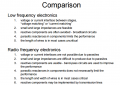# low frequency electronics vs radio frequency electronics

#### Ali Alkhudri

Joined Nov 3, 2015
19
Hello brothers
I started with a course called Amplifier design and I do not understand some issues
Here is a comparison between low freq. and radio freq. electronics ...
Can you tell me some examples of radio freq. electronics?
What current interface between stages mean?
what reactive components mean?
What parasitic reactances mean?
Why the length of wires is critical in radio freq. components?
What transmission lines mean?Thank you

#### bertus

Joined Apr 5, 2008
22,133
Hello,

First of all:
What is the definition of "low frequency"?
And what is the definition of "Radio frequency"?
Radio frequency can be anything from 10 kHz upto many GHz's.

Bertus

#### Ali Alkhudri

Joined Nov 3, 2015
19
Hello,

First of all:
What is the definition of "low frequency"?
And what is the definition of "Radio frequency"?
Radio frequency can be anything from 10 kHz upto many GHz's.

Bertus
thanks
do you know Why the length of wires is critical in radio freq. components?

#### sirch2

Joined Jan 21, 2013
1,037
Look at the equations for capacitive reactance and inductive reactance. You will see that capacitive reactance decreases with frequency and inductive reactance increases with frequency.

Now a capacitor is, in its simplest form, a pair of parallel conductors separated by an insulator this means that any two conductors close together (such as a wire and a PCB) have a capacitance, at low frequency the reactive loss is negligible but at very high frequencies it matters. This is a parasitic loss.

The opposite applies to inductors.

#### Ali Alkhudri

Joined Nov 3, 2015
19
Look at the equations for capacitive reactance and inductive reactance. You will see that capacitive reactance decreases with frequency and inductive reactance increases with frequency.

Now a capacitor is, in its simplest form, a pair of parallel conductors separated by an insulator this means that any two conductors close together (such as a wire and a PCB) have a capacitance, at low frequency the reactive loss is negligible but at very high frequencies it matters. This is a parasitic loss.

The opposite applies to inductors.
thank you#### crutschow

Joined Mar 14, 2008
31,493
At low frequencies parasitic (stray) capacitance and inductance reactance generally have only a small effect on circuit operation.
Because the reactance of inductance and capacitance changes with frequency, these become more important as you increase the frequency, at some point will cause a serious deviation of the circuit operation from the intended. Thus for high frequency circuits you need to lay out the circuit to minimize these stray reactances.

Here's a reference on transmission lines.